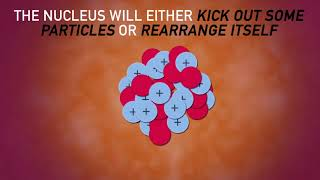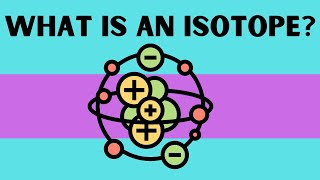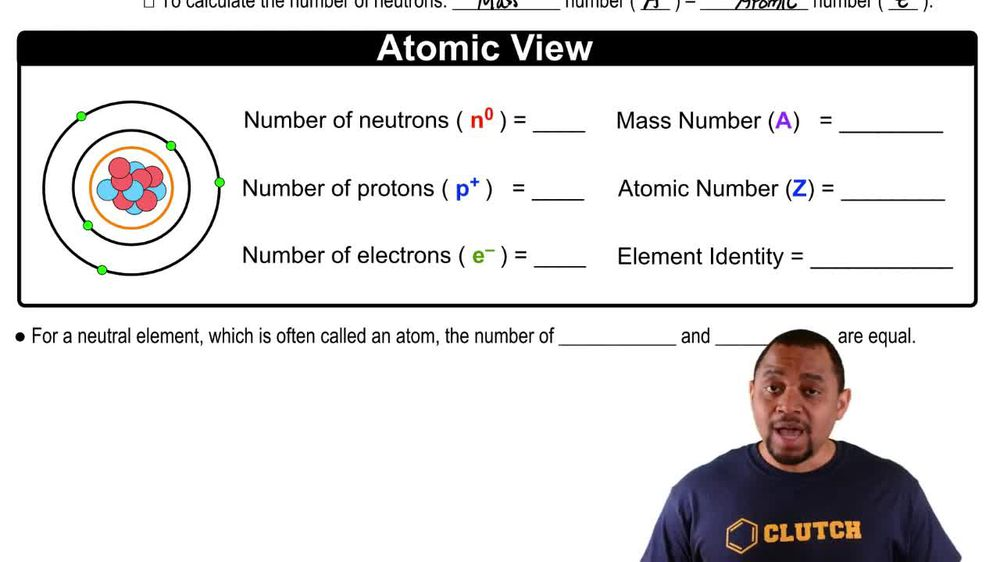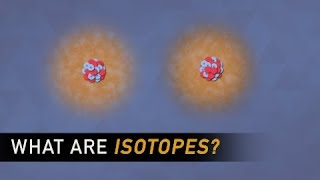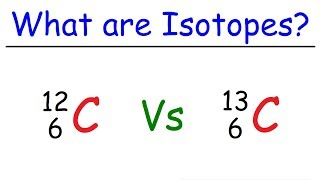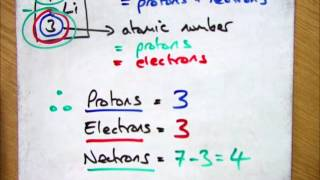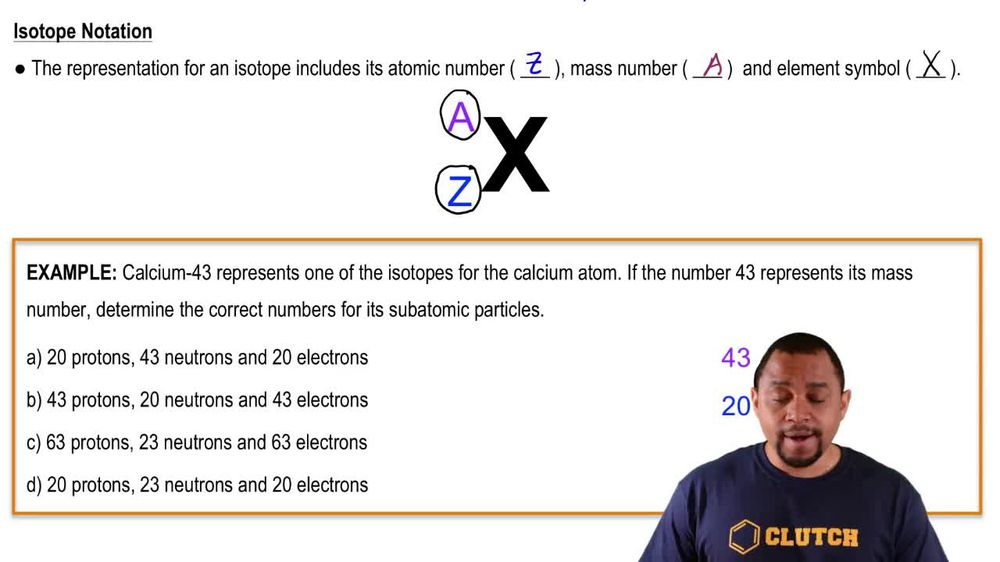Start typing, then use the up and down arrows to select an option from the list.
1. 2. Atoms & Elements2. Isotopes
Problem

# Write isotopic symbols in the form AZX for each isotope. c. the potassium isotope with 21 neutrons

Relevant Solution1m
Play a video:
Hey everyone in this example, we need to identify the isotope symbol of our described isotope with 41 neutrons. We first need to recall a few things. The first being that the a superscript on the left hand side of our isotope symbol here is representative of our mass number and we can find this by taking our Z value which we should recall. Z represents our atomic number of our isotope and subtracting that. We're sorry, adding that to our number of neutrons. So let's go ahead and find germanium on our periodic tables. When we look on our periodic tables, we find Germany in Germany um in group for A And we see that it corresponds to the atomic number 32. So we would say that Z is equal to 32. So now focusing on our mass number, which is our symbol for a. We go ahead and we can take this Atomic number And add it to our given number of neutrons. So we know that N is equal to 41 because that is what is given to us. So we can say 32 plus 41 is equal to our mass number here. So this gives us a total of 73. And so to complete our isotope symbol, we can say therefore we have an isotope symbol where we replace the X here with our isotope Romania. We replaced the a with what we just calculated above for mass number 73 And we fill in 32 for Z our atomic number. And so this will complete the chemical isotope symbol for our Germanium isotope with 41 neutrons. This is our final answer. If you have any questions, leave them down below, and I will see everyone in the next practice video.